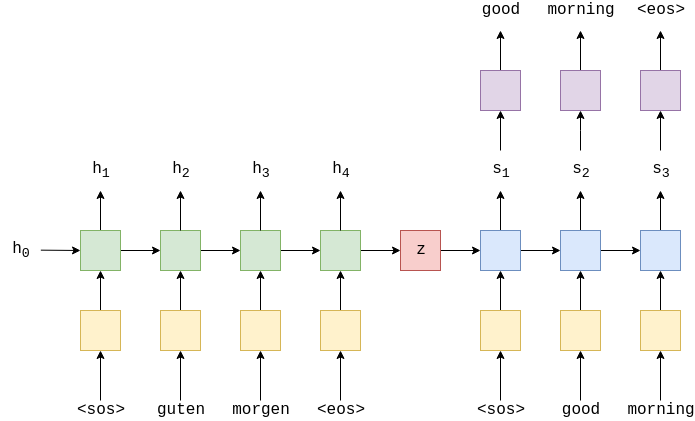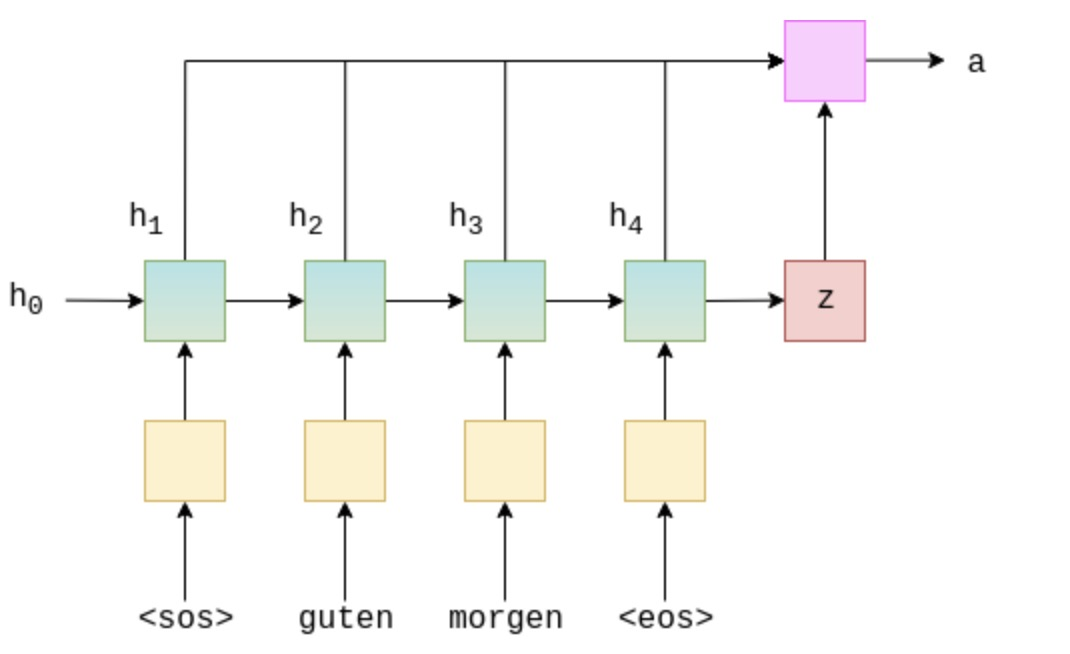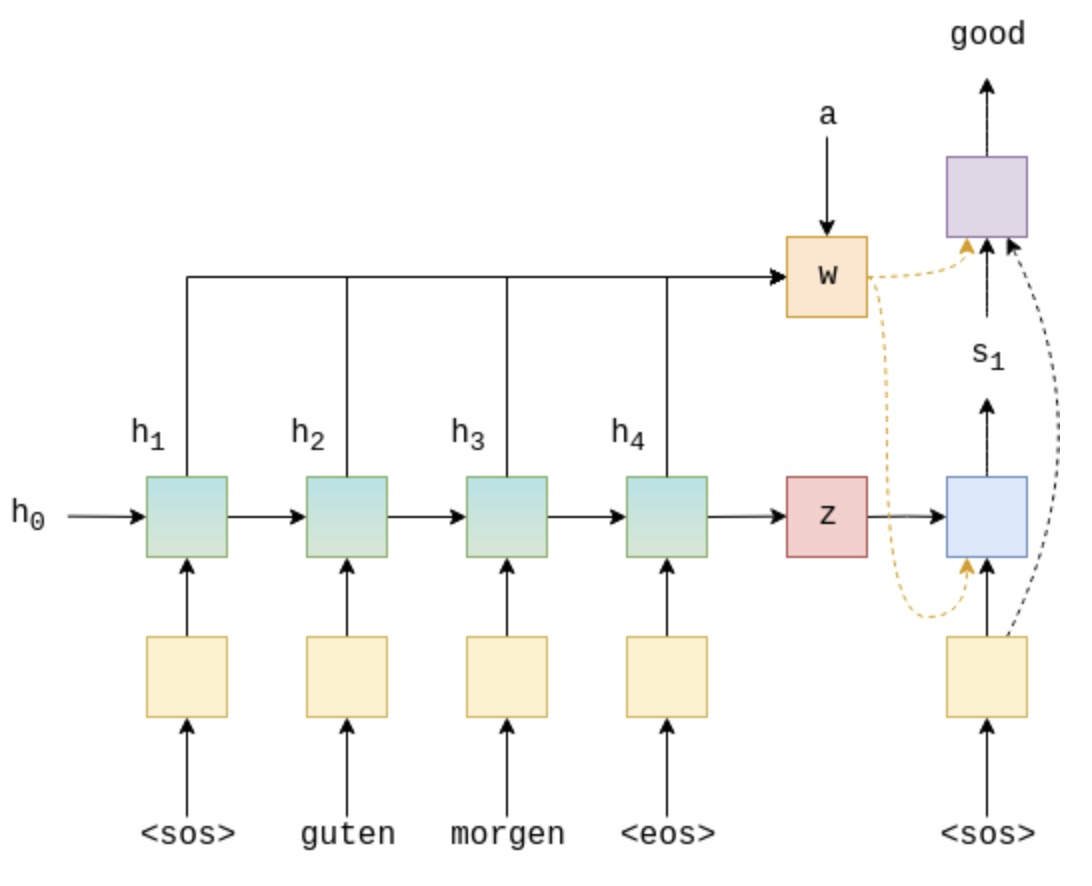# Seq2Seq模型: 从理论到实践(二)## （一）信息压缩问题class Decoder(nn.Module):
def __init__(self, output_dim, emb_dim, hid_dim, dropout):
"""
:param output_dim: 目标词汇表的大小
:param emb_dim: 词向量大小
:param hid_dim: GRU 隐层神经元个数
:param dropout:
"""
super().__init__()
self.hid_dim = hid_dim
self.output_dim = output_dim

self.embedding = nn.Embedding(output_dim, emb_dim)

# context vector 与 hid_dim 大小一样
# GRU 的输入为 concat [context vector, embedding vector]
self.rnn = nn.GRU(emb_dim + hid_dim, hid_dim)
# 分类层输入为 concat [context vector, embedding vector, hidden dim]
self.fc_out = nn.Linear(emb_dim + hid_dim * 2, output_dim)

self.dropout = nn.Dropout(dropout)

def forward(self, x, hidden, context):
"""

:param x: [batch_size]
:param hidden:
:param context:
:return:
"""
#  x = [1, batch size]
x = x.unsqueeze(0)

# 目标token embedding
# embedded = [1, batch size, emb dim]
embedded = self.dropout(self.embedding(x))

# 将embed vector 与 context concat
# emb_con = [1, batch size, emb dim + hid dim]
emb_con = torch.cat((embedded, context), dim = 2)

# GRU 输出
output, hidden = self.rnn(emb_con, hidden)

# cat 当前的隐状态、embedding vector和 context vector
# output = [batch size, emb dim + hid dim * 2]
output = torch.cat((embedded.squeeze(0), hidden.squeeze(0), context.squeeze(0)),
dim = 1)

# 分类
# prediction = [batch size, output dim]
prediction = self.fc_out(output)

return prediction, hidden



## （二） Attention seq2seq

$$a_t=attn(s _{t-1}, H)$$• 编码器部分对输入序列进行编码，输出每个时间步的hidden state作为attention 需要的输入和最后一个时间步的状态作为context vector
• 解码器的每一个时间步, 首先根据上一个时间步得到的hidden state 和编码器所有的hidden state $W$ 得到attention的权值，将 $W$ 加权后和embedded vector输入解码器.$$Attention(Q,K,V)=softmax(match(Q,K)) * V$$